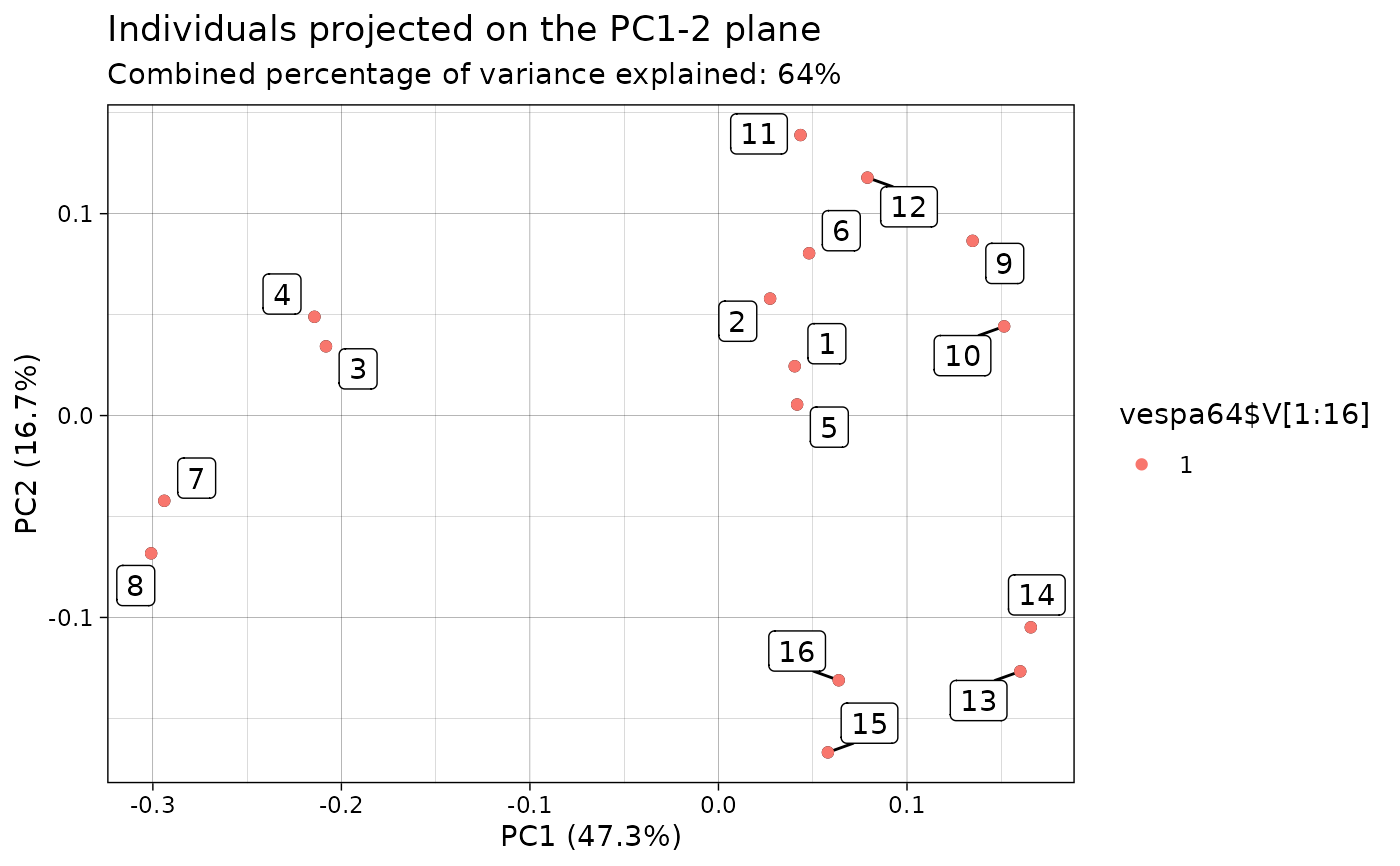This function creates a visualization of the results of the PCA applied on a sample of QTS and returns the corresponding ggplot2::ggplot object which enable further customization of the plot.

## Usage

# S3 method for prcomp_qts
autoplot(object, what = "PC1", ...)

## Arguments

object

An object of class prcomp_qts as produced by the prcomp.qts_sample() method.

what

A string specifying what kind of visualization the user wants to perform. Choices are words starting with PC and ending with a PC number (in which case the mean QTS is displayed along with its perturbations due to the required PC) or scores (in which case individuals are projected on the required plane). Defaults to PC1.

...

If what = "PC?", the user can specify whether to plot the QTS in the tangent space or in the original space by providing a boolean argument original_space which defaults to TRUE. If what = "scores", the user can specify the plane onto which the individuals will be projected by providing a length-2 integer vector argument plane which defaults to 1:2.

## Value

A ggplot2::ggplot object.

## Examples

df <- as_qts_sample(vespa64$igp[1:16]) res_pca <- prcomp(df) #> ℹ The maximum number of principal component is 15. # Plot the data points in a PC plane # And color points according to a categorical variable p <- ggplot2::autoplot(res_pca, what = "scores") #> The plane length-2 integer vector argument is not specified. Defaulting to #> 1:2. p + ggplot2::geom_point(ggplot2::aes(color = vespa64$V[1:16]))